# Active Band Pass Filter Circuit Diagram and Its Frequency Response

## Active Band Pass Filter

The Active Band Pass Filter is  a frequency selective filter  used in electronic systems to separate a signal at one particular frequency, or a range of signals that lie within a certain “band” of frequencies from signals at all other frequencies.

This band or range of frequencies is set between two cut-off or corner frequency points labelled the “lower frequency” ( ƒL ) and the “higher frequency” ( ƒH ) while attenuating any signals outside of these two points.

A simple Active Band Pass Filter can be easily made by cascading together a single Low pass Filter with a single High pass Filter as shown below.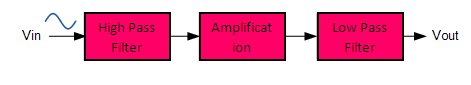The cut-off frequency of the LPF is higher than the cut-off frequency of the HPF and the difference between the frequencies at the -3dB point will determine the “bandwidth” of the band pass filter .

For example, suppose we have a band pass filter whose -3dB cut-off points are set at 200Hz and 600Hz. Then the bandwidth of the filter would be given as: Bandwidth (BW) = 600 – 200 = 400Hz.

One way of making a very simple Active Band Pass Filter is to connect the basic passive high and low pass filters to an amplifying op-amp circuit as  below .

### Active Band Pass Filter Circuit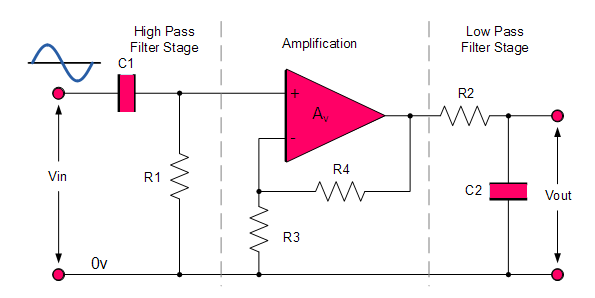This cascading together of a low and a high pass passive filters produces a low “Q-factor” type filter circuit which has a wide pass band.

The first stage of the filter will be the high pass stage that uses the capacitor to block any DC biasing from the source.

This design has the advantage of producing a relatively flat asymmetrical pass band frequency response with one half representing the high pass response and the other half representing low pass response as shown below.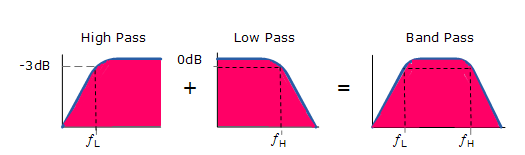The higher cut off frequency ( ƒH ) as well as the lower cut off  frequency  ( ƒL ) are calculated the same as before in the standard first-order low and high pass filter circuits.

In this filter circuit, a reasonable separation is required between the two cut-off points to prevent any interaction between the low pass and high pass stages.

The amplifier also provides isolation between the two stages and defines the overall voltage gain of the circuit.

### Frequency Response of Active Band Pass Filter

The frequency response and phase shift for an active band pass filter will be shown below .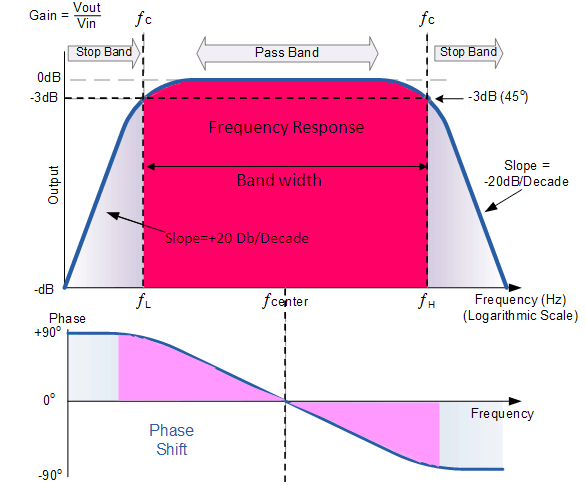### Resonant Frequency Point

An active band pass filter is a 2nd Order type filter since it has “two” reactive components (two capacitors) within its circuit design.

Due to these two reactive components, the filter will have a peak response or Resonant Frequencyƒr ) at its “center frequency”, ƒc.

The center frequency is generally calculated as being the geometric mean of the two -3dB frequencies between the upper and the lower cut-off points and the resonant frequency is given as: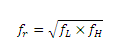where  f=  resonant or Center Frequency

ƒL is the lower -3dB cut-off frequency point

ƒH is the upper -3db cut-off frequency point

### The “Q” or Quality Factor

In a Band Pass Filter circuit, the overall width of the actual pass band between the upper and lower -3dB corner points of the filter determines the Quality Factor or Q-point of the circuit.

This Q Factor is a measure of how “Selective” or “Un-selective” the band pass filter is towards a given spread of frequencies.

The lower the value of the Q factor the wider is the bandwidth of the filter and consequently the higher the Q factor the narrower and more “selective” is the filter.

The Quality Factor, Q of the filter is also known as the alpha-peak frequency and given as :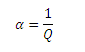The “Q” of a band pass filter is the ratio of the Resonant Frequency, ( ƒr ) to the Bandwidth, ( BW ) between the upper and lower -3dB frequencies and is given as: